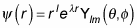##### Quantum Physics For Dummies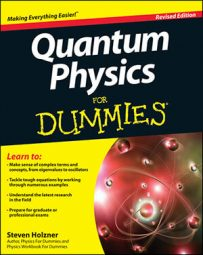When you apply the quantum mechanical Schrödinger equation for a hydrogen atom, you need to put together the solutions for small r and large r. The Schrödinger equation gives you a solution to the radial Schrödinger equation for a hydrogen atom as follows: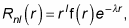where f(r) is some as-yet-undetermined function of r. Your next task is to determine f(r), which you do by substituting this equation into the radial Schrödinger equation, giving you the following: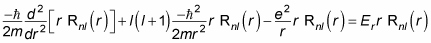Performing the substitution gives you the following differential equation: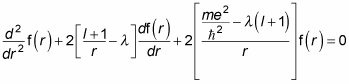Quite a differential equation, eh? But just sit back and relax — you solve it with a power series, which is a common way of solving differential equations. Here’s the power-series form of f(r) to use: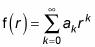Substituting the preceding equation into the one before it gives you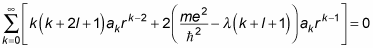Changing the index of the second term from k to k – 1 gives you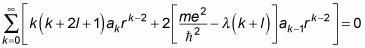Because each term in this series has to be zero, you have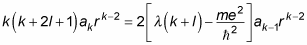Dividing by rk–2 gives you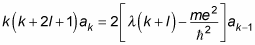This equation gives the recurrence relation of the infinite series,That is, if you have one coefficient, you can get the next one using this equation. What does that buy you? Well, take a look at the ratio of ak/ak–1: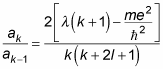Here’s what this ratio approaches as k goes to infinity: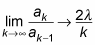This resembles the expansion for ex, which is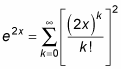As for e2x, the ratio of successive terms is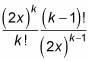And in the limit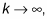the ratio of successive expansion coefficients of e2x approaches 2/k: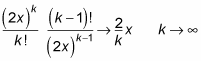That’s the case for e2x. For f(r), you haveComparing these two equations, it’s apparent that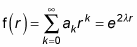The radial wave function, Rnl(r), looks like this: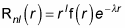where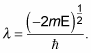Plugging the form you have for f(r),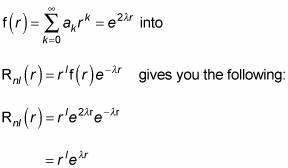Okay, should you be overjoyed? Well, no. Here’s what the wave function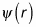looks like: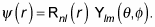And substituting in your form of Rnl(r) from this equation gives you